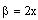4.
____________O___________
Again, Mathcad will only give answers that lie in the range of the inverse sine function, i. e.,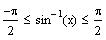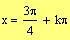==>==>Divide by 2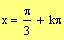or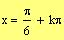==>
Use unit circle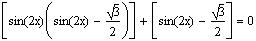Take sin(2x) as a common factor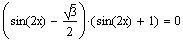Takeas a common factor
Thus,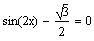or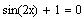Use the zero product property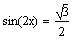or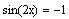==>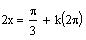orSolve for t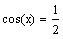orSubstitute cos(x) for t==>or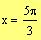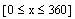==>Given
To find the other angle (in Quadrant IV) whose cosine is 1/3, we subtract 70.5 from 360: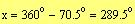Given that cos-1(1/3) = 70.5o , solve for x in the interval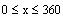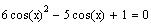Solution:
Let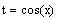Substitute t for cos(x)
==>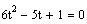Factoror(Which property did we use?)or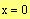The solutions are:
If we substitute x = 180o in the original equation, we get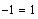. Similarly for the value
x = 270o. Thus, x = 180o and x = 270o are not solutions.
However, because we squared both sides in the process of the solution, one has to be careful about introducing "extraneous" solutions (solutions that will not satisfy the original equation).or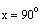ororUse zero product propertyor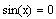Divide by 2
Math 002-041
Major Quiz 8 Solution
Nov. 29, 2004
Solve the following problems for x:
1.Solution:Square both sides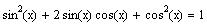Expand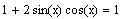Use the identity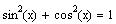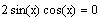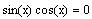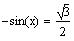Using the fact that the sine function is odd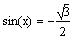The sine function is negative in Quadrant III and IV. Using the method of the unit circle (see Lecture Notes web page), we have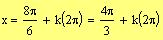or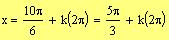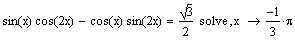Remark
Mathcad returned only one value because it used the inverse sine function (sin-1 ). Recall that the range of sin-1(x) is betweenand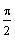.
_____________O_____________
3.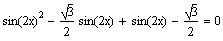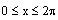Solution: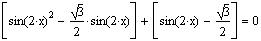Group to take common factors
or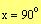RemarkThe solution is given in radians which is the same as the one we obtained in degrees.
____________O_____________
2.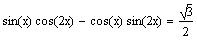Solution: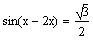Using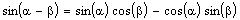Where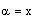and